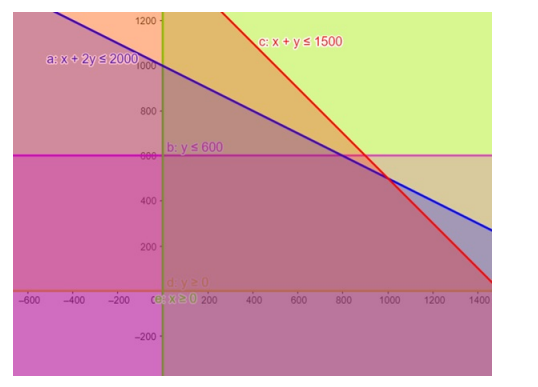# Solve the given inequalities

Question:

Solve the given inequalities $x+2 y \leq 2000, x+y \leq 1500, y \leq 600, x \geq 0, y \geq 0$ graphically in two dimensional plane.

Solution:

The graphical representation of $x+2 y \leq 2000, x+y \leq 1500$

$y \leq 600, y \geq 0, x \geq 0$ is given by common region in the figure below.

$x+2 y \leq 2000 \ldots \ldots$ (1)

$x+y \leq 1500 \ldots \ldots$ (2)

$x \geq 0 \ldots \ldots$ (3)

$y \geq 0 \ldots \ldots$ (4)

$y \leq 600 \ldots \ldots$ (5)

Inequality (1) represents the region below line $x+2 y=2000$ (including the line $x+2 y=2000$ ).

Inequality (2) represents the region below line $x+y=1500$ (including the line $x+y=1500$ ).

Inequality (3) represents the region in front of line $x=0$ (including the line $x=0$ ).

Inequality (4) represents the region above line $y=0$ (including the line $y=0$ ).

Inequality (5) represents the region below line $y=600$ (including the line $y=600$ )

Therefore,every point in the common shaded region including the points on the respective lines represents the solution for the given inequalities.

This can be represented as follows,